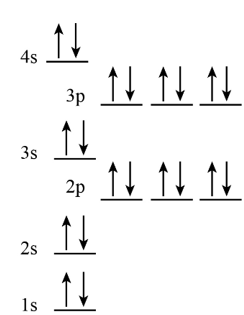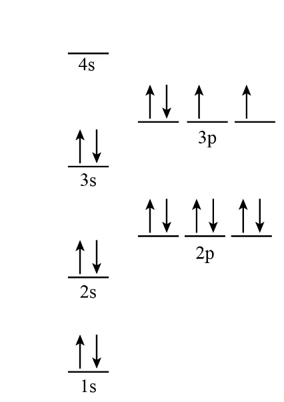# Write orbital notation, electron configurations, and noble gas electron configuration for each of...

## Question:

Write orbital notation, electron configurations, and noble gas electron configuration for each of the following elements.

(a) {eq}\rm Ca {/eq}.

(b) {eq}\rm S {/eq}.

## Electronic configuration

Electronic configuration is used to represent number of electrons present in atomic orbital of an atom. While noble gas electronic configuration includes the last noble gas prior to that atom and followed by the configuration of the leftover electrons.

(a)

The given element is calcium. The electronic configuration of calcium is{eq}\rm 1{s^2}2{s^2}2{p^6}3{s^2}3{p^6}4{s^2} {/eq} and the noble gas electronic configuration is {eq}\rm [Ar] 4{s^2} {/eq}.The orbital notation for calcium is shown below:(b)

The given element is sulfur. The electronic configuration of sulfur is {eq}\rm 1{s^2}2{s^2}2{p^6}3{s^2}3{p^4} {/eq}. The noble gas electronic configuration is {eq}\rm [Ne] 3{s^2}3{p^4} {/eq}. The orbital notation for sulfur is shown below:D-Block Elements: Properties & Electron Configuration

from

Chapter 6 / Lesson 9
21K

In chemistry, the d-block elements are the transition elements. Learn what this means by exploring the properties and electron configuration of the d-block elements. Understand how electrons act in d-block elements and review how transition metals are used.